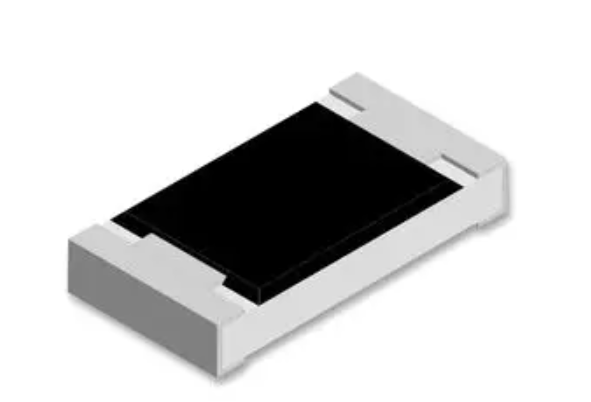< img src="https://mc.yandex.ru/watch/53493796" style="position:absolute; left:-9999px;" alt="" />
Need Help?## CRGCQ0805J100K

CRGCQ0805J100K is a type of surface mount general purpose resistor that is commonly used in electronic circuits. Here’s a breakdown of the code: “CRGC” indicates the manufacturer of the resistor, which in this case is Vishay Dale. “Q” refers to the product family of the resistor. “0805” indicates the package size of the resistor, which […]

## Description

CRGCQ0805J100K is a type of surface mount general purpose resistor that is commonly used in electronic circuits.

Here’s a breakdown of the code:

• “CRGC” indicates the manufacturer of the resistor, which in this case is Vishay Dale.
• “Q” refers to the product family of the resistor.
• “0805” indicates the package size of the resistor, which is a common size for surface mount resistors. The dimensions are 0.08 x 0.05 inches.
• “J” indicates the tolerance of the resistor. J indicates a tolerance of +/- 5%.
• “100K” indicates the resistance value of the resistor, which is 100 kilohms.

So in summary, the CRGCQ0805J100K is a surface mount resistor with a 100 kilohm resistance and a tolerance of +/- 5%.

## CRGCQ0805J100K Feature

1. Resistance value: The “J100K” in the part number indicates that the resistance value is 100 kΩ (kiloohms).
2. Power rating: This resistor has a power rating of 125 mW (milliwatts), which means that it can handle up to 125 milliwatts of power without overheating.
3. Tolerance: The tolerance of this resistor is ±5%, which means that the actual resistance value can vary by up to 5% from the stated value of 100 kΩ.
4. Operating temperature range: The CRGCQ0805J100K resistor can operate in temperatures ranging from -55°C to 155°C, making it suitable for use in a wide range of environments.
5. Size: This resistor has a package size of 0805, which means that it measures 0.08 inches by 0.05 inches.

### FAQ

Q:What is a surface mount chip resistor?

A:A surface mount chip resistor is a small electronic component that is designed to be mounted directly onto a printed circuit board (PCB). They are smaller and more compact than traditional through-hole resistors, making them ideal for use in compact electronic devices.

Q:What does the “0805” in the part number mean?

A:The “0805” in the part number refers to the package size of the resistor. In this case, the package size is 0805, which means that the resistor measures 0.08 inches by 0.05 inches.

Q:What is the tolerance of this resistor?

A:The tolerance of the CRGCQ0805J100K resistor is ±5%. This means that the actual resistance value can vary by up to 5% from the stated value of 100 kΩ.

Q:What is the power rating of this resistor?

A:The power rating of the CRGCQ0805J100K resistor is 125 mW (milliwatts). This means that it can handle up to 125 milliwatts of power without overheating.

SEND EMAIL TO US